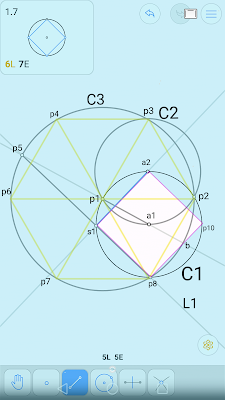## Tuesday, 14 November 2017

### Inscribe square within circle. Geometric construction.

```From question on Euclidea geometry app.

Given: circle C1 and points a1 at centre of circle and a2 on circle.
Construct: square inscribed in C1 with one corner at a2.
Must do it using only 7 elementary moves(i.e. draw circle or line)

Question is answered here:
https://math.stackexchange.com/questions/1587861/inscribing-square-in-circle-in-just-seven-compass-and-straightedge-steps#

I found that inscribing hexagon (and it's 6 equilateral triangles) in the big circle helped me to understand how the construction works. 60 degree and 30 degree and 15 degree angle divisions are key.F

```
```

It is still difficult (for me!) to break it down and prove how it works. Anyway. I have tried. It could perhaps be simplified.

Given: circle C1 and points a1 at centre of circle and a2 on circle.
Construct: square inscribed in C1 with one corner at a2.

1. Draw circle C2 with centre at a2 and a1 on circle (same radius as C1).
Call the points where C1 and C2 intersect p1 and p2.

line lengths |a1p1| = |p1a2| = |a1a2| = radius of C1,C2
so little triangles a1a2p1 and a1a2p2 (not shown) are equilateral (60 degree angles)
6 of these triangles could be inscribed into C2 giving a smaller hexagon
this smaller hexagon gives us point p3 and proves triangle p1p2p3 is equilateral.

2. Draw big circle C3 with centre p1 and passing through p2.

We know Tri p1p2p3 is equilateral, we can also construct equilateral triangle p1p2p8 and construct hexagon p2p3p4p6p7p8
This construction also shows that p8 and p3 where big circle C3 intersects C2 and C1 align (vertical in diagram) with a1 and a2.

3. Draw Line a1p1 extended, this gives us points p5 and b where it intersects C3

We want to construct side of square line p8s1(s1 not marked leftmost point on square) at 15 degrees to p8p1
```
```

```
```

The squares' sides are at angles of 45 degrees from horizontal. Line p8p1 on equilateral triangle is 60 degrees from horizontal. 15 degrees between them.

Line a1p1 bisects triangle p1p2p8 (as it does also to triangle p1p4p6 and hexagon) as it is side of little triangle a1a2p1

so

Consider Triangle p1p5p8

We can see
Lines p1p5 = p1p8 = radius of big circle

so

So point s1 is on line p8p5 where it intersects C1

4. Draw Line p5p8 giving square side s1p8

Line p5b is diameter of circle C3

Angle Thales' theorem

```
This means Line p8b extended to p10 is another side of the square.

5. Draw Line p8b extended to p10 where it intersects C1 giving another side of square p8p10

6. Draw side a2p10

7. Draw side a2s1

That's it.

Interesting to note the angles in the circle segments. And the lengths of the circle segments. Circles C1 (and C2) give a sixth of circumference of circle C3.

Interesting also the angles around point a2 (and around p8):

360 - 240 - 90 = 360 - 330 = 30 degrees
So
```
```
```
```